HomeTallyPrimeSales ProcessRound Off Invoice and Ledger Values
Explore Categories

# Round Off Invoice and Ledger Values

When you record an invoice, the total invoice value will not be rounded off automatically. To round off the value of the invoice or the value of tax ledger in the invoice, you can use the round off feature in TallyPrime.

## Invoice Round Off – Using predefined ledger

When you record an invoice, you may want to maintain a ledger exclusively to round off the invoice value. For this, you can create an Invoice Rounding ledger in TallyPrime.

1. Create a ledger for round off.
1. Create the ledger under Indirect Expenses.
2. Type of Ledger – Select Invoice Rounding.
3. In Rounding method, select the appropriate rounding method.
• Not Applicable – Keep the invoice value as is.
• Downward Rounding – Round off the invoice value to the nearest lower number that is a multiple of the rounding limit specified.
For example, if the invoice value is 125.60, the invoice value will be rounded off to 125 (when the Rounding limit is set to 1), and the round off ledger will have the value (-)0.60.
• Normal Rounding – Round off the invoice value to the:
• Nearest lower number that is a multiple of the rounding limit specified if the decimal value is less than 0.5. For example, if the invoice value is 125.30, the value will be rounded off to 125, and the round off ledger will have the value (-)0.30.
• Nearest higher number that is a multiple of the rounding limit specified if the decimal value is more than or equal to 0.5. For example, if the value is 125.60, it will be rounded off to 126, and the round off ledger will have the value 0.40 (when the Rounding limit is set to 1).
• Upward Rounding – Round off the invoice value to the nearest higher number that is a multiple of the rounding limit specified. For example, if the invoice value is 125.30, the value will be rounded off to 126, and the round off ledger will have the value 0.70 (when the Rounding limit is set to 1).
4. For Rounding limit, enter the number to calculate the nearest number as a multiple of this number to round off. For example, if you mention 5, the invoice value will be rounded off to the nearest number that is a multiple of 5.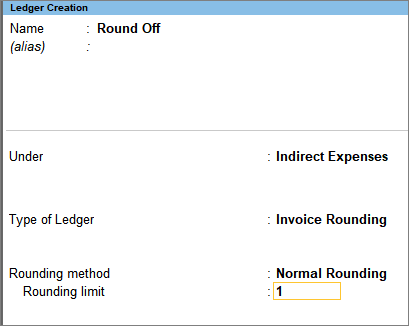5. Accept the ledger. As always, you can press Ctrl+A to save.
2. Use the round off ledger in sales invoice. The invoice value will be rounded off, and the difference in value due to rounding off will be displayed as a positive or negative number.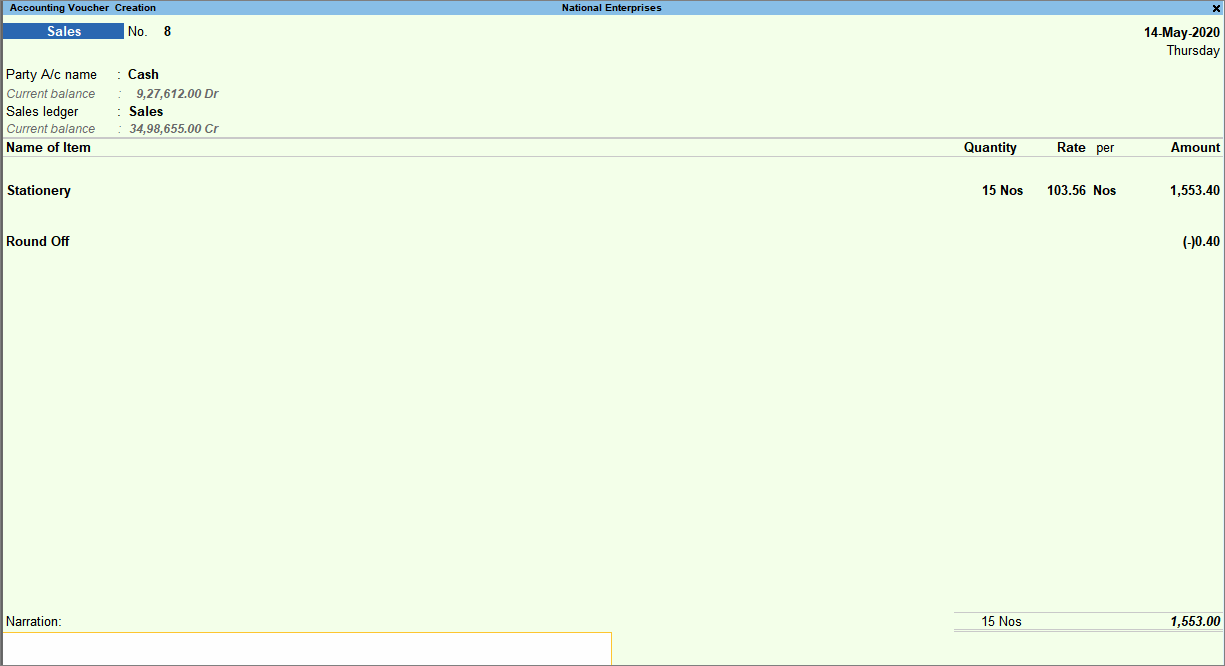3. Accept the invoice. As always, you can press Ctrl+A to save.

You can use this procedure to round off the invoice value.

## Invoice Round Off – Using Voucher Class

You may want the round off ledger to automatically appear in the invoice, to avoid selecting it every time you record transaction. For this, you can create voucher class in TallyPrime with the round off ledger, and use this voucher class to automatically display the round off ledger in the invoice.

1. Create a voucher class for sales.
1. Gateway of Tally > Alter > Voucher Type > type or select Sales > and press Enter.
Alternatively, Alt+G (Go To) > Alter Master > Voucher Type > type or select Sales > and press Enter.
2. Enter a name for the voucher class under Name of Class.
3. Press Enter to view the Voucher Type Class screen.
4. Under Additional Accounting Entries, select a ledger created under Indirect Expenses for accounting invoice round off. In this ledger, set Use ledger for auto calculation of to Not Applicable.
5. For the Type of Calculation, select As Total Amount Rounding.
6. In Rounding method, select the appropriate rounding method.
• Not Applicable – Keep the invoice value as is.
• Downward Rounding – Round off the invoice value to the nearest lower number that is a multiple of the rounding limit specified. For example, if the invoice value is 125.60, the invoice value will be rounded off to 125 (when the Rounding limit is set to 1), and the round off ledger will have the value (-)0.60.
• Normal Rounding – Round off the invoice value to the:
• Nearest lower number that is a multiple of the rounding limit specified if the decimal value is less than 0.5. For example, if the invoice value is 125.30, the value will be rounded off to 125, and the round off ledger will have the value (-)0.30.
• Nearest higher number that is a multiple of the rounding limit specified if the decimal value is more than or equal to 0.5. For example, if the value is 125.60, it will be rounded off to 126, and the round off ledger will have the value 0.40 (when the Rounding limit is set to 1).
• Upward Rounding – Round off the invoice value to the nearest higher number that is a multiple of the rounding limit specified. For example, if the invoice value is 125.30, the value will be rounded off to 126, and the round off ledger will have the value 0.70 (when the Rounding limit is set to 1).
7. For Rounding Limit, enter the number to calculate the nearest number as a multiple of this number to round off. For example, if you mention 5, the invoice value will be rounded off to the nearest number that is a multiple of 5.
8. Remove if Zero – Set it to Yes to remove the ledger from the invoice if the ledger value is zero.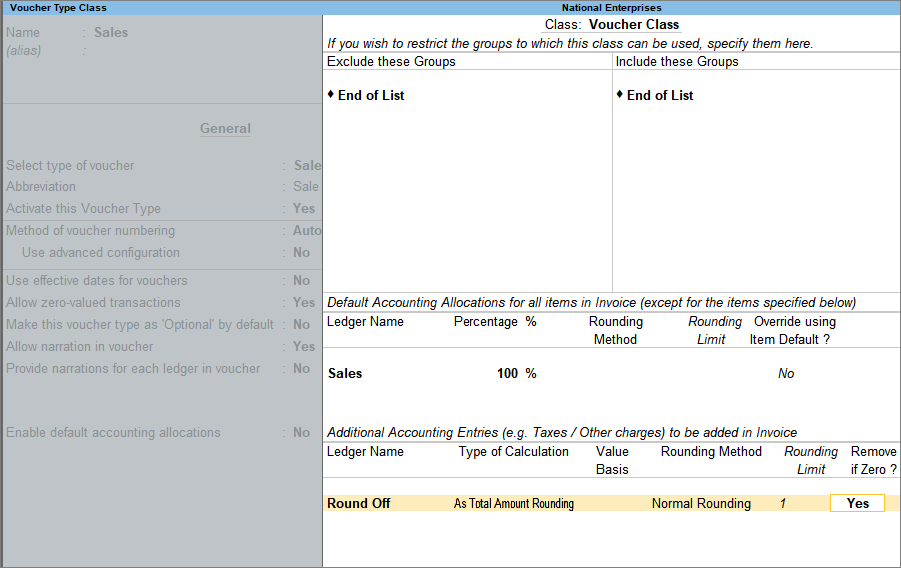9. Accept the screen and the sales voucher type. As always, you can press Ctrl+A to save.
2. Use the voucher class in sales invoice.
1. Gateway of Tally > Vouchers > F8 (Sales) > select the voucher class.
Alternatively, press Alt+G (Go To) > Create Voucher > F8 (Sales) > select the voucher class.
The round off ledger appears automatically.
2. Enter the sales details.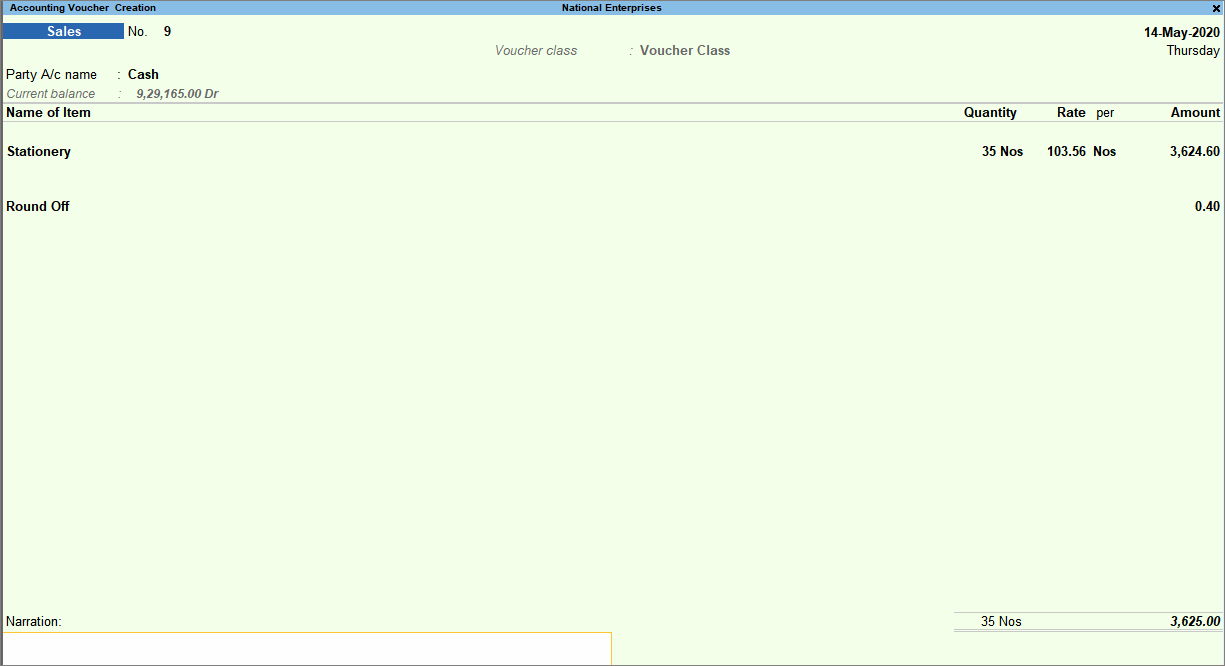3. Accept the invoice. As always, you can press Ctrl+A to save.

You can use this procedure to use voucher class, to round off the invoice value.

## Round Off only the ledger value

When you record an invoice, you may want to round off the value of the tax ledger. For this you can use the round off feature in TallyPrime.

1. Alter the ledger created under Duties & Taxes.
1. For the Rounding method, select the appropriate rounding method.
• Not Applicable – Keep the invoice value as is.
• Downward Rounding – Round off the invoice value to the nearest lower number that is a multiple of the rounding limit specified. For example, if the invoice value is 125.60, the invoice value will be rounded off to 125 (when the Rounding limit is set to 1), and the round off ledger will have the value (-)0.60.
• Normal Rounding -Round off the invoice value to the:
• Nearest lower number that is a multiple of the rounding limit specified if the decimal value is less than 0.5. For example, if the invoice value is 125.30, the value will be rounded off to 125, and the round off ledger will have the value (-)0.30.
• Nearest higher number that is a multiple of the rounding limit specified if the decimal value is more than or equal to 0.5. For example, if the value is 125.60, it will be rounded off to 126, and the round off ledger will have the value 0.40 (when the Rounding limit is set to 1).
• Upward Rounding – Round off the invoice value to the nearest higher number that is a multiple of the rounding limit specified. For example, if the invoice value is 125.30, the value will be rounded off to 126, and the round off ledger will have the value 0.70 (when the Rounding limit is set to 1).
2. For Rounding limit, enter the number to calculate the nearest number as a multiple of this number to round off. For example, if you mention 5, the invoice value will be rounded off to the nearest number that is a multiple of 5.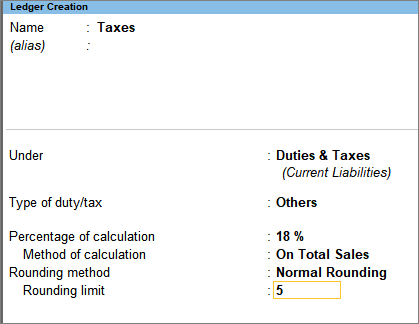3. Accept the ledger. As always, you can press Ctrl+A to save.
2. In the sales voucher, select the ledger. The ledger value will be rounded off as per the rounding method and limit.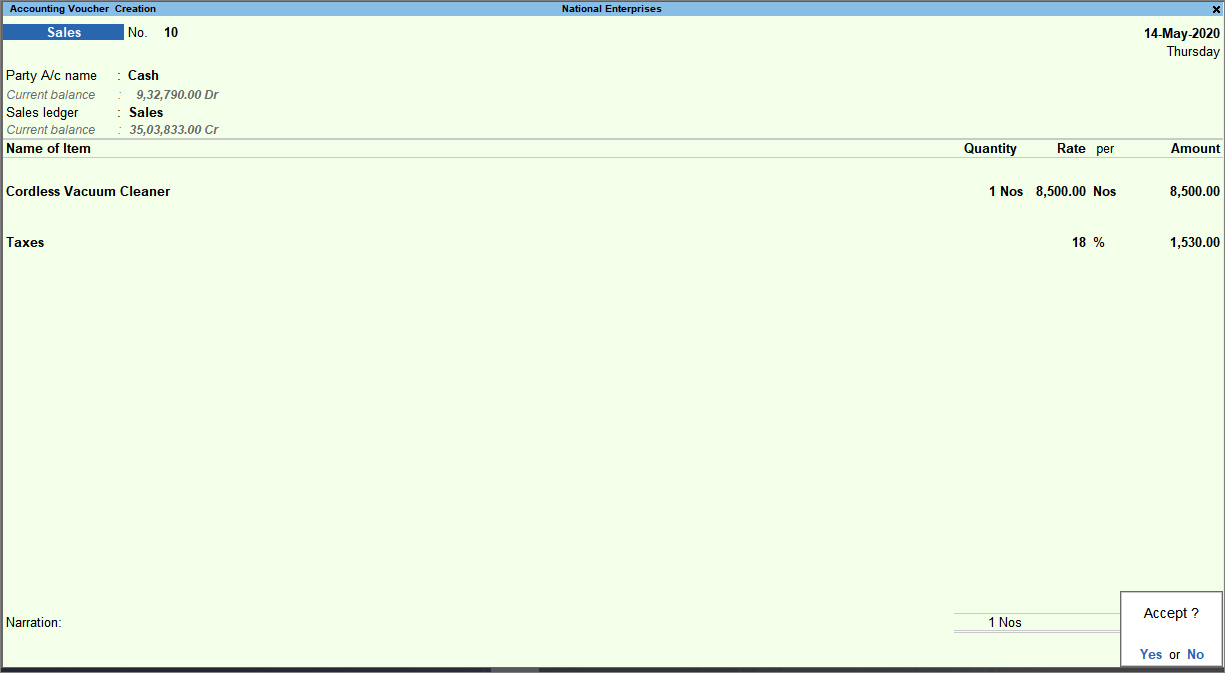In the above example, if the rounding method was set to Not Applicable, the tax amount would be 4,788.87. As the rounding method is set to Normal Rounding, with Rounding limit as 5, the tax amount is calculated as 4,790.00.

You can use this procedure to round off the ledger value in sales invoice.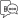Helpful?